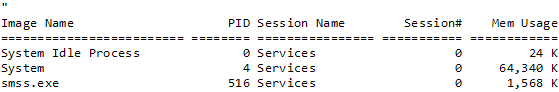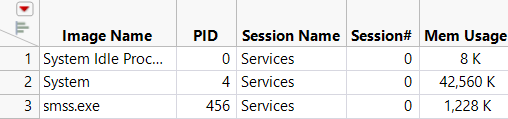Publication date: 11/10/2021

## Run External Programs

Run Program() enables you to control a program that uses standard input and standard output. Line-mode programs read commands from stdin and write answers to stdout. cmd.exe, the Windows command prompt, is one example. You can also use it to launch GUI programs, such as Windows Notepad.

`Run Program(`
`	Executable( "cmd.exe" ),`
`	Options( {"/c", "TaskList\!n"} ),`
`	Read Function( "text" ) // returns all text`
`);`

The preceding script writes the Windows task list and details to the log.

Figure 8.3 Log OutputYou can also print the task list directly without using cmd.exe and then import the task list into a data table.

`blob = Run Program(`
`	Executable( "tasklist.exe" ),`
`	Read Function( "blob" )`
`);`
` `
`Open(`
`	blob,`
`	Columns(`
`		Column( "Image Name", Character, "Nominal" ),`
`		Column(`
`			"PID",`
`			Numeric,`
`			"Continuous",`
`			Format( "Best", 10 )`
`		),`
`		Column( "Session Name", Character, "Nominal" ),`
`		Column(`
`			"Session#",`
`			Numeric,`
`			"Continuous",`
`			Format( "Best", 10 )`
`		),`
`		Column( "Mem Usage", Character, "Nominal" ),`
`		Omitted Column( . )`
`	),`
`	Import Settings(`
`		Fixed Column Widths( 26, 9, 17, 12, 12, 63 ),`
`		Labels( 1 ),`
`		Column Names Start( 2 ),`
`		Data Starts( 4 )`
`	)`
`);`

Figure 8.4 Imported Data from Run Program()Note: If you use Run Program() to launch a GUI program, JMP is inoperable until you close the program.